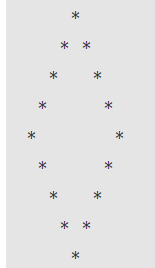Related Tags

javascript
communitycreator

# How to create a hollow diamond pattern in JavaScript

Ayush Trivedi

### Overview

In this shot, we will learn how to create a hollow diamond star pattern using JavaScript. This pattern is more complex than the simple diamond patterns. Combining the logic of the upside and downside hollow triangle is the simplest way to make a hollow diamond design.

A hollow diamond star pattern looks like the image below:### Code

let n = 5;
for (let i = 1; i <= n; i++) {
for (let j = n; j > i; j--) {
process.stdout.write(' ');
}
for (let k = 0; k < i * 2 - 1; k++) {
if (k === 0 || k === 2 * i - 2) {
process.stdout.write('*');
}
else {
process.stdout.write(' ');
}
}
console.log();
}

for (let i = 1; i <= n - 1; i++) {
for (let j = 0; j < i; j++) {
process.stdout.write(' ');
}
for (let k = (n - i) * 2 - 1; k >= 1; k--) {
if (k === 1 || k === (n - i) * 2 - 1) {
process.stdout.write('*');
}
else {
process.stdout.write(' ');
}
}
console.log();
}

### Code explanation

• In line 1, we initialize a number that is the height (rows) of the upside triangle (i.e., 5) and one less for the downside triangle (i.e., 4), so total rows (height) for the diamond is 9.

#### Upside triangle (From lines 2-15)

• In line 2, we have this outer for loop, which will iterate over the height of the upside triangle (which is 5, here n=5).
• In line 3, we have the first nested for loop, which will print spaces n-i times. For example, if i=3, then the number of spaces for the third row is 2, for i=4 is 1, and so on.
• In line 6, we have the second nested for loop to print * at the border of the triangle and the spaces in between.
• In line 7, we have the if condition to check if the iteration is for the border of the diamond, and print * there.
• In line 10, we have the else condition. If the iteration is for inside the diamond, then it will print spaces.

#### Downside triangle (from lines 17-30)

• In line 17, we have our second outer for loop, which will iterate for one less than the height of the downside triangle (which is n-1 = 4 times, here n=5).
• In line 18, we have the first nested for loop, which will print spaces i times. For example, if i=1, then the number of spaces for the first row is 1, for i=4 is 4, and so on.
• In line 21, we have the second nested for loop to print * at the border of the triangle and spaces in between.
• In line 22, we have the if condition to check if the iteration is for the border of the diamond, and print * there.
• In line 25, we have the else condition. If the iteration is for inside the diamond, then it will print spaces.

### Output:

We have completed the task to create a hollow diamond star pattern in JavaScript.

RELATED TAGS

javascript
communitycreator

CONTRIBUTOR

Ayush Trivedi
RELATED COURSES

View all Courses

Keep Exploring

Learn in-demand tech skills in half the time